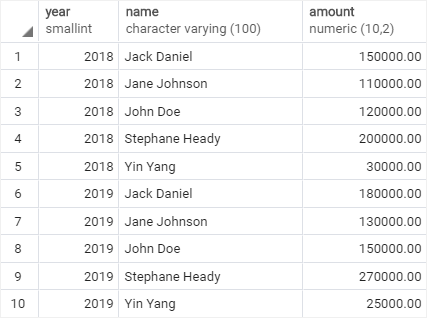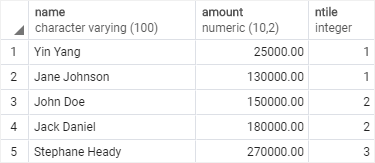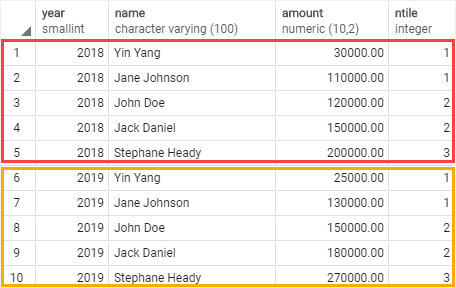# PostgreSQL Tutorial: NTILE Function

August 7, 2023

Summary: in this tutorial, you will learn how to use the PostgreSQL `NTILE()` function to divide ordered rows in the partition into a specified number of ranked buckets.

## Introduction to PostgreSQL `NTILE()` function

The PostgreSQL `NTILE()` function allows you to divide ordered rows in the partition into a specified number of ranked groups as equal size as possible. These ranked groups are called buckets.

The `NTILE()` function assigns each group a bucket number starting from 1. For each row in a group, the `NTILE()` function assigns a bucket number representing the group to which the row belongs.

The syntax of the `NTILE()` function is as follows:

``````NTILE(buckets) OVER (
[PARTITION BY partition_expression, ... ]
[ORDER BY sort_expression [ASC | DESC], ...]
)
``````

Let’s examine the syntax in detail:

### `buckets`

The `buckets` represents the number of ranked groups. It can be a number or an expression that evaluates to a positive integer value (greater than 0) for each partition. The `buckets` must not be nullable.

### `PARTITION BY`

The `PARTITION BY` clause distributes rows into partitions to which the function is applied.

The `PARTITION BY` clause is optional. If you skip it, the function treats the whole result set as a single partition.

### `ORDER BY`

The `ORDER BY` clause sorts rows in each partition to which the function is applied.

The `ORDER BY` clause is optional. However, you should always use the `ORDER BY` clause to get an expected result.

Note that if the number of rows is not divisible by the `buckets`, the `NTILE()` function returns groups of two sizes with the difference by one. The bigger groups always come before the smaller groups in the order specified by the `ORDER BY` clause.

## PostgreSQL `NTILE()` function examples

Let’s take some examples of using the `NTILE()` function.

We’ll use the `sales_stats` table created in the `CUME_DIST()` function tutorial to demonstrate the `NTILE()` function.

``````SELECT
year,
name,
amount
FROM
actual_sales
ORDER BY
year, name;
``````### 1) Using PostgreSQL `NTILE()` function over a result set example

This example uses the `NTILE()` function to distribute rows into 3 buckets:

``````SELECT
name,
amount,
NTILE(3) OVER(
ORDER BY amount
)
FROM
sales_stats
WHERE
year = 2019;
``````

Here is the output:### 2) Using PostgreSQL `NTILE()` function over a partition example

This example uses the `NTILE()` function to divide rows in the `sales_stats` table into two partitions and 3 buckets for each:

``````SELECT
name,
amount,
NTILE(3) OVER(
PARTITION BY year
ORDER BY amount
)
FROM
sales_stats;
``````

Here is the result set:In this tutorial, you have learned how to use the PostgreSQL `NTILE()` function to distribute ordered rows within a partition into a specified number of ranked groups.